# The SIM2D Procedure

#### Theoretical Development

It is a simple matter to produce an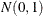random number, and by stacking krandom numbers in a column vector, you can obtain a vector with independent standard normal components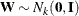. The meaning of the terms independence and randomness in the context of a deterministic algorithm required for the generation of these numbers is subtle; see Knuth (1981, Chapter 3) for details.

Rather than, what is required is the generation of a vector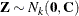—that is,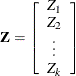with covariance matrix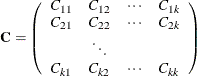If the covariance matrix is symmetric and positive definite, it has a Cholesky root
such that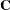can be factored as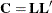whereis lower triangular. See Ralston and Rabinowitz (1978, Chapter 9, Section 3-3) for details. This vectorcan be generated by the transformation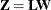. Here is where the assumption of a Gaussian SRF is crucial. When, thenis also Gaussian. The mean ofis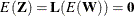and the variance is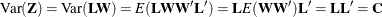Consider now an SRF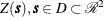, with spatial covariance function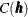. Fix locations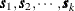, and letdenote the random vector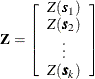with corresponding covariance matrix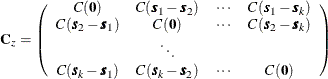Since this covariance matrix is symmetric and positive definite, it has a Cholesky root, and the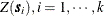, can be simulated as described previously. This is how the SIM2D procedure implements unconditional simulation in the zero-mean case. More generally,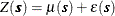where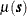is a quadratic form in the coordinates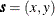and the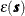is an SRF that has the same covariance matrix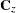as previously. In this case, the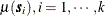, is computed once and added to the simulated vector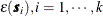, for each realization.

For a conditional simulation, this distribution ofmust be conditioned on the observed data. The relevant general result concerning conditional distributions of multivariate normal random variables is the following. Let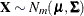, where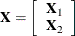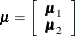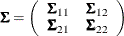The subvector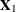is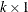,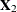is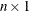,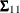is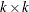,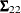is, and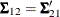is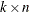, with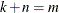. The full vectoris partitioned into two subvectors,and, and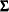is similarly partitioned into covariances and cross covariances.

With this notation, the distribution ofconditioned on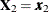is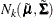, with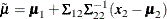and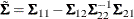See Searle (1971, pp. 46–47) for details. The correspondence with the conditional spatial simulation problem is as follows. Let the coordinates of the observed data points be denoted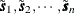, with values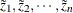. Let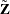denote the random vector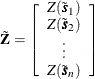The random vectorcorresponds to, whilecorresponds to. Then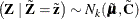as in the previous distribution. The matrix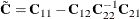is again positive definite, so a Cholesky factorization can be performed.

The dimension n foris simply the number of nonmissing observations for the VAR= variable; the valuesare the values of this variable. The coordinatesare also found in the DATA= data set, with the variables that correspond to the x and y coordinates identified in the COORDINATES statement. Note: All VAR= variables use the same set of conditioning coordinates; this fixes the matrix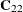for all simulations.

The dimension k foris the number of grid points specified in the GRID statement. Since there is a single GRID statement, this fixes the matrix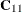for all simulations. Similarly,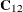is fixed.

The Cholesky factorization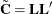is computed once, as is the mean correction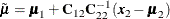The means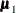and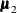are computed using the grid coordinates, the data coordinates, and the quadratic form specification from the MEAN statement. The simulation is now performed exactly as in the unconditional case. A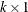vector of independent standardrandom variables is generated and multiplied by, and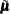is added to the transformed vector. This is repeated N times, where N is the value specified for the NR= option.